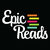# Would These Characters Be On Your Naughty or Nice List?

## We love morally questionable characters—a lot. In fact, we might be a little bit biased because we like them too much. So we're turning to you, readers, to let us know what you think of some of them.Created By Epic Reads
On Dec 11, 2018
Calculating results
Calculating results
Calculating results
Calculating results
Calculating results
Calculating results
Calculating results
Calculating results
Calculating results
Calculating results
Calculating results
Calculating results